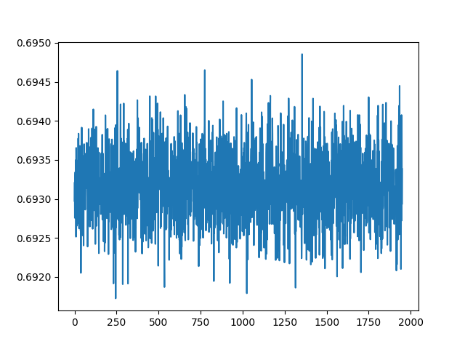# Pytorch: Loss of Conv-neural-network not decreasing, instead obsoleting

I have a convolutional neural network in vgg architecture “style” (down below) to classify images if there is a cat on the picture, or a dog. My training set contains 25000 images cropped to 256px each side. I tried different learning rates, different loss functions and much more but my loss keeps fluctuating between 0.692 and 0.694, but it will not decrease…

``````    normalize = transforms.Normalize(
mean=[0.485, 0.456, 0.406],
std=[0.229, 0.224, 0.225]
)

transform = transforms.Compose([
transforms.Resize(256),
transforms.CenterCrop(256),
transforms.ToTensor(),
normalize
])

# Output = [isDog, isCat]
train_data_list = []
train_data = []
target_list = []
plotlist = []
train_files = listdir("data/catsdogs/train/")

global train_data_list
global train_data
global target_list

amount = len(listdir("data/catsdogs/train/"))
current = 0

for i in range(amount):
r = random.randint(0, len(train_files) - 1)
file = train_files[r]
train_files.remove(file)

img = Image.open("data/catsdogs/train/" + file)
img_tensor = transform(img)  # (3, 256, 256)

isCat = 1 if 'cat' in file else 0
isDog = 1 if 'dog' in file else 0
target = [isCat, isDog]

train_data_list.append(img_tensor)
target_list.append(target)

if len(train_data_list) >= 64:
train_data.append((torch.stack(train_data_list), target_list))
train_data_list = []
target_list = []

current = current + 1
print("Loaded: {:.1f}%".format(current * 100 / amount))

class Network(nn.Module):
def __init__(self):
super(Network, self).__init__()
self.conv1 = nn.Conv2d(3, 16, kernel_size=3, stride=1, padding=1)
self.conv2 = nn.Conv2d(16, 16, kernel_size=3, stride=1, padding=1)

self.conv3 = nn.Conv2d(16, 32, kernel_size=3, stride=1, padding=1)
self.conv4 = nn.Conv2d(32, 32, kernel_size=3, stride=1, padding=1)

self.conv5 = nn.Conv2d(32, 64, kernel_size=3, stride=1, padding=1)
self.conv6 = nn.Conv2d(64, 64, kernel_size=3, stride=1, padding=1)

self.dropout = nn.Dropout2d()
self.relu = nn.ReLU(inplace=True)
self.pool = nn.MaxPool2d(kernel_size=3, stride=3, padding=0, dilation=1, ceil_mode=False)

self.fc1 = nn.Linear(5184, 1296)
self.fc2 = nn.Linear(1296, 2)

def forward(self, x):
# Block 1
x = self.conv1(x)
x = self.relu(x)

x = self.conv2(x)
x = self.relu(x)

x = self.pool(x)

# Block 2
x = self.conv3(x)
x = self.relu(x)

x = self.conv4(x)
x = self.relu(x)

x = self.pool(x)

# Block 3
x = self.conv5(x)
x = self.relu(x)

x = self.conv6(x)
x = self.relu(x)

x = self.pool(x)

x = x.view(-1, 5184)

x = F.relu(self.fc1(x))
x = self.dropout(x)
x = self.fc2(x)

model = Network()
model = model.cuda()

optimizer = optim.SGD(model.parameters(), lr=0.0001, weight_decay=0.0016)

def train(epoch):
global optimizer

model.train()
batch_id = 0
for data, target in train_data:
data = data.cuda()
target = torch.Tensor(target).cuda()

data = Variable(data)
target = Variable(target)

out = model(data)
criterion = F.binary_cross_entropy

loss = criterion(out, target)
loss.backward()

optimizer.step()

plotlist.append(loss)

print('Train Epoch: {},  {:.0f}% ,\tLoss: {:.6f}'.format(
epoch, 100. * batch_id / len(train_data), loss.item()
))
batch_id = batch_id + 1

for epoch in range(25):
train(epoch)

plt.plot(plotlist)
plt.show()
plt.ylabel("Loss")
plt.savefig("lossPlot.png")

``````

If I increase the learning rate, the fluctuation get heavier… For example for lr = 0.1 between 0.5 and 0.7
Also here is a plot of my loss over 5 iterations with lr 0.0001:Is this `Variable` function the `torch.autograd.Variable` which is deprecated? Your data and target need not be Trainable. Also just for future reference if you want any tensor to be trainable just set its `required_grad` attribute as `True` or simply `a.required_grad_()` assuming `a` is a `torch` tensor.

I couldn’t help but notice that the data is normalized with strange numbers, why not standard normalize it (0, 1)?
Additionally, you could try training with different optimizers too for example `Adam` maybe?

1 Like

It looks like Variable was the right call here.
The normalization values of the images were preferred in another tutorial, which had used the same images. Also I switched to Adam, it gives a slight improvement… But still, my loss is heavily obsoleting… Even if it goes down:The loss curves look fine to me though! Now you need to tune your hyperparameters to make the results better and maybe adding more regularization, augmentation, some architectural changes and what not…

1 Like

I’ll try, whish me luck thanks xD
Update: with slight changes and taking the mean of every iteration: